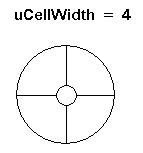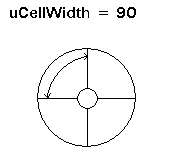﻿ CellWidth Property (PixelateCommand) | Leadtools.ImageProcessing.SpecialEffects | Raster, Medical, Document Help
←Select platform
In This Topic ▼

# CellWidth Property

Summary

Gets or sets a value that represents the width of a rectangular cell.

Syntax
C#
VB
C++
``public int CellWidth { get; set; } ``
````  `
`Public Property CellWidth As Integer ````
````public: `
`property int CellWidth { `
`   int get(); `
`   void set (    int ); `
`} ````

#### Property Value

Value that represents the width of a rectangular cell, the number of rectangular cells present across the width of the image, the number of cells around the center point of a circular cell, or the length in degrees of each cell around the center point of a circular cell, based on the flags set in the Flags property.

 If the Flags property contains: Then the CellWidth property contains: And the range of possible values for the CellWidth property is: PixelateCommandFlags.Radial | PixelateCommandFlags.WidthFrequency the number of cells around the CenterPoint property. That is, the circle around the center point is divided into equal parts based on the value of the CellWidth property, as shown below:1 to 360. The circle can be divided into anywhere from 1 to 360 equal parts. PixelateCommandFlags.Radial | PixelateCommandFlags.WidthPeriod the size, in degrees of the cells around the center point That is, the circle around the center point is divided into cells, based on the number of degrees in the CellWidth property, as shown below:1 to 360. The circle can be divided into cells of 1 degree to 360 degrees. PixelateCommandFlags.Rectangle | PixelateCommandFlags.WidthFrequency the number of cells present across the width of the image. 1 to the image width, if there is no region. If there is a region, then the range of values is just the region width. PixelateCommandFlags.Rectangle | PixelateCommandFlags.WidthPeriod | the width of each rectangular cell, in pixels. 1 to the image width, if there is no region. If there is a region, then the range of values is just the region width.

Example

Refer to PixelateCommand.

Requirements